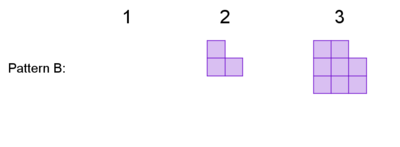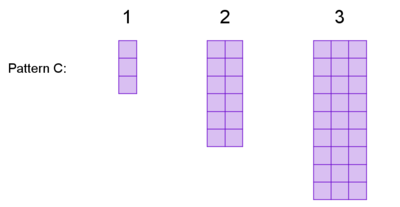##### Engage your students with effective distance learning resources. ACCESS RESOURCES>>

Alignments to Content Standards: A-REI.B.4.b

The first three steps of three visual patterns are shown below.Here are functions that define how many tiles are in step $n$, in no particular order: $$f(n)=3n^2$$ $$g(n)=n^2+4$$ $$h(n)=n^2-1$$

1. Decide which function defines which pattern.
2. One of these patterns has a step with $432$ tiles in it, one has a step with $195$ tiles in it, and one of these patterns has a step with $404$ tiles in it. Decide which is which, find the step that contains that number of tiles, and explain how you know.
3. Describe and justify the steps to solve this equation for $x$. $$ax^2+c=d$$

## IM Commentary

This task belongs to a series of three tasks that presents students with a sequence of tile figures with the property that the $n$-th figure in the sequence has $f(n)$ tiles, for some quadratic function $f$. Students are asked to analyze the functions in the context of the tile sequence, a process which involves manipulating the quadratic expressions into different forms (identifying square roots at first, then completing the square in the third task.) With solving quadratics, there can be an impulse to put everything in standard form and just use the quadratic formula. However, seeing structure is emphasized in the standards because of how it connects and helps in understanding many foundational concepts, and these tasks develop the ability to see structure when working with quadratic expressions and equations.

That the domain of these quadratic functions is the set of positive integers provides an interesting wrinkle which students might not be used to thinking about in the setting of quadratic functions. The functions roughly increase in complexity through the three tasks, with the intent that the techniques learned in each will be used and expanded in the subsequent tasks. The other tasks in the sequence are Quadratic Sequence 2 and Quadratic Sequence 3.

Part (a) has many possible points of entry. For example, students might evaluate each function at $n=1$, or they might analyze the way the patterns grow visually. For example, each step of Pattern A consists of $n^2$ tiles with four tiles tacked on, and each step of Pattern B consists of $n^2$ tiles with one tile removed. (Here is a good primer on what that conversation might look like.)

Ideally, students will have had some experience working with visual patterns prior to this task. If not, students might benefit from additional questions to familiarize themselves with the idea. For example, they could be asked to "draw the next step" for one or more patterns. Part (a) could be scaffolded with more specific instructions; for example, students could be asked to evaluate $g(3)$, find that $g(3) = 13$, and conclude that this means the pattern defined by g(n) must have 13 tiles in step 3.

The purpose of part (b) is to motivate solving a quadratic equation where the variable of interest, $x$, can be isolated by undoing operations.

In part (c), students have an opportunity to express regularity in repeated reasoning (MP.8) by applying these same undoing operations to an equation to isolate a variable of interest in terms of other variables.

This task was inspired by visualpatterns.org.

## Solution

1. $f(n)$ defines Pattern C, $g(n)$ defines Pattern A, and $h(n)$ defines Pattern B. There are many ways to determine this. You could evaluate each function for $n=1$. For example, $f(1)=3$. This tells you that the first step of the pattern defined by $f$ has 3 tiles, so $f$ must go with Pattern C. Another method would be to see how each pattern grows visually. For example, step $n$ of Pattern A has a square of $n \times n$ tiles with 4 tiles tacked on, so $g(n)=n^2+4$ makes sense.
2. Pattern A has 404 tiles in step 20, Pattern B has 195 tiles in step 14, and Pattern C has 432 tiles in step 12. We need to see which function has an integer solution for each number of given tiles. For example, to check whether $f(n)$ has a step with $432$ tiles, we can write $$3n^2=432$$ $$n^2=144$$ which has a solution of $n=12$. So, step $12$ of Pattern C has $432$ tiles. However, when we check whether Pattern A can have $195$ tiles and write $$n^2+4=195$$ and then $$n^2=191,$$but this does not have integer solutions. So Pattern A does not have any step with $195$ tiles, so by elimination, Pattern B must be the one with  $195$ tiles. Indeed we find that the equation $$n^2-1=195$$ $$n^2=196$$ has a solution of $n=14$. So Pattern B has $195$ tiles in step $14$. Finally, a solution to $n^2+4=404$ is $n=20$, so step $20$ of Pattern A must have $404$ tiles.
3. In figuring out part (b), we did some simple reasoning about equations to undo operations and find solutions. Now we apply those techniques generally. $$ax^2+c=d$$ $$ax^2=d-c$$ $$x^2=\frac{d-c}{a}$$ $$x=\pm\sqrt{\frac{d-c}{a}}$$ For tiles and steps, solutions can only take positive values, but generally, solutions could be the positive or negative value of the square root.